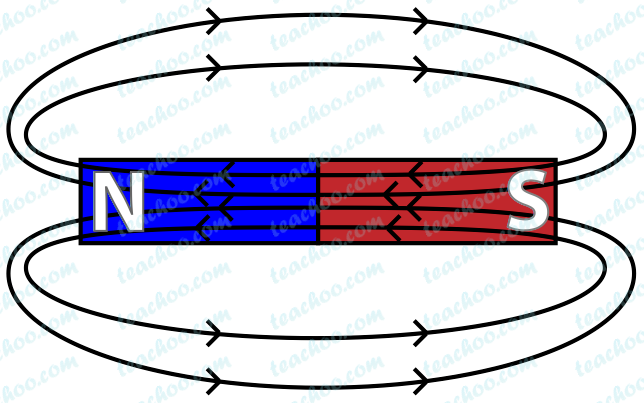Teachoo Questions

Class 10
Chapter 13 Class 10 - Magnetic Effects of Electric Current (Term 2)

The password to open the file is -    teachooisbest

## Why is magnetism at the center of a bar magnet zero?

Magnetic field lines in a bar magnet looks likeThe magnetic field at the center of the bar magnet is not 0, but weak.

Infact, magnetic field is minimum at the center

Explanation

We know that,

the more magnetic field lines in region, the more magnetic field.

At the center of a bar magnet, magnetic field lines are parallel. So, magnetic field is less there

## What is an armature? Why is it used?

An armature is a loop of insulated copper wire in the form of a rectangle used in an electric motor.

Function of armature:

An armature enhances the power of the motor and induces motion

## Name devices in which permanent magnets are used.

Devices in which permanent magnets are used

• Speakers
• Generators
• Microphones
• Compass

## If the number of circular current carrying coils are  (i) doubled (ii) halved, then how will the magnetic field produced by it changed?

We know that,

Magnetic field of a current coil is directly proportional to the number of turns/coils.

(a) when number of coils are doubled

Magnetic field of a current coil is directly proportional to the number of turns/coils.

Since number of coils are doubled, magnetic field produced will also double.

(b) when number of coils are halved

Magnetic field of a current coil is directly proportional to the number of turns/coils.

Since number of coils are halved, magnetic field produced will also be halved.

## What is the usual current rating of the fuse wire in the line to feed

1. Lights and fans
2. Appliances of 2kW or more power